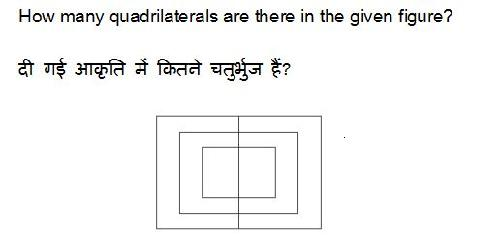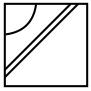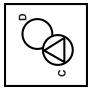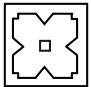# SSC MTS 2017 RE-EXAM PAPER : Held on 04-OCTOBER-2017 Shift-1 (GENERAL INTELLIGENCE AND REASONING)

QID : 11) 42
2) 43
3) 44
4) 48
QID : 2 -1) 8
2) 9
3) 10
4) 12
QID : 3 -1) 21, 55, 67, 41
2) 79, 55, 87, 41
3) 21, 31, 67, 57
4) 79, 32, 67, 57
Correct Answer: 79, 55, 87, 41
QID : 4 -1)2)3)4)QID : 5 -1)2)3)4)QID : 6 -1)2)3)4)QID : 7 -1)2)3)4)QID : 8 - If '–' means '+', '×' means '÷', '÷' means '–' and '+' means '×', then

9 × 6 + 36 – 4 = ?
1) 58
2) 38
3) 40
4) 32
QID : 9 - If 5 + 3 + 1 = 15 and 6 + 1 + 4 = 24, then 4 + 3 + 0 = ?
1) 4
2) 12
3) 0
4) 8
QID : 10 - Arrange the given words in the sequence in which they occur in the dictionary.
1. Jelly
2. Jenny
3. Jewel
4. Jocks
5. Jiffy
1) 12345
2) 12354
3) 13254
4) 21354
QID : 11 - In the following question, select the missing number from the given series.
121, 4, 144, 9, 169, 16, 196, ?
1) 225
2) 36
3) 25
4) 40
QID : 12 - A series is given with one term missing. Select the correct alternative from the given ones that will complete the series.
W, T, Q, N, ?
1) J
2) K
3) L
4) M
QID : 13 - In the following question, select the odd word from the given alternatives.
1) Grass
2) Plant
3) Tree
4) Land

QID : 14 - In the following question, select the odd number pair from the given alternatives.

1) 7 – 52
2) 11 – 124
3) 12 – 147
4) 14 – 201
QID : 15 - In the following question, select the odd letters from the given alternatives.
1) CDEH
2) JKLO
3) RSTW
4) EFGK
QID : 16 - Identify the diagram that best represents the relationship among the given classes.
Biology, Science, Subject
1)2)3)4)QID : 17 - In the following question, select the related word from the given alternatives.
Metal : Ore : : Paper : ?
1) Flour
2) Pulp
3) Brick
4) Seed
QID : 18 - In the following question, select the related number from the given alternatives.
1 : 8 : : 216 : ?
1) 343
2) 125
3) 64
4) 729
QID : 19 - In the following question, select the related letters from the given alternatives.
FA : CC : : PX : ?
1) KY
2) LY
3) MZ
4) KZ
QID : 20 - In the following question, select the word which cannot be formed using the letters of the given word.
TOURNAMENT
1) TORMENT
2) RENT
3) TOUR
4) TRAP
QID : 21 - Pointing to a man, Heena said "he is the only son of wife of my father". How is the man related to Heena?
1) Grandfather
2) Husband
3) Brother
4) Son
QID : 22 - Nayan remembers his examinations starts after 15th August, while her mother remembers that his examinations starts before 18th August. On which date of August does his examinations start?
1) 16
2) 17
3) 16 or 17
4) 17 or 18
QID : 23 - In the following question below are given some statements followed by some conclusions. Taking the given statements to be true even if they seem to be at variance from commonly known facts, read all the conclusions and then decide which of the given conclusion logically follows the given statements.
Statements:
I. Some yellow are small.
II. All small are red.
Conclusions:
I. Some red are yellow.
II. Some small are yellow.
1) Only conclusion (I) follows.
2) Only conclusion (II) follows.
3) Neither conclusion (I) nor conclusion (II) follows.
4) Both conclusion follow.